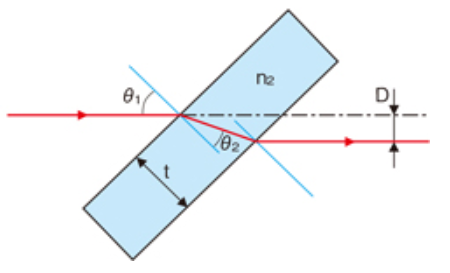# Distance Between The Incident and the Refraction

This calculator will help you calcaulate the distance between the incident and the refraction light after light travels through a planar plate## $D$= $d$sin($\theta_1$-$\theta_2$)

Incident Refractive Index $n_1$;
Refraction Refractive Index $n_2$;
Incident angle $\theta_1$;
Refraction angle $\theta_2$;
The Thickness of The Plate $d$

Input
degree (°)
mm

Output
mm